GeeksforGeeks App
Open AppBrowser
Continue

# Class 10 NCERT Solutions- Chapter 6 Triangles – Exercise 6.3 | Set 2

### Question 11. In the following figure, E is a point on side CB produced of an isosceles triangle ABC with AB = AC. If AD ⊥ BC and EF ⊥ AC, prove that ΔABD ~ ΔECF.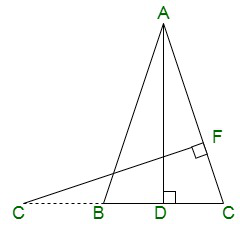Solution:

Given, ABC is an isosceles triangle.

Since, the sides of an isosceles triangle are equal, we have,

∴ AB = AC

⇒ ∠ABD = ∠ECF

In ΔABD and ΔECF,

Since, each of the following angles are 90°.

By AA similarity criterion, we have,

∴ ΔABD ~ ΔECF

### Question 12. Sides AB and BC and median AD of a triangle ABC are respectively proportional to sides PQ and QR and median PM of ΔPQR (see Fig 6.41). Show that ΔABC ~ ΔPQR.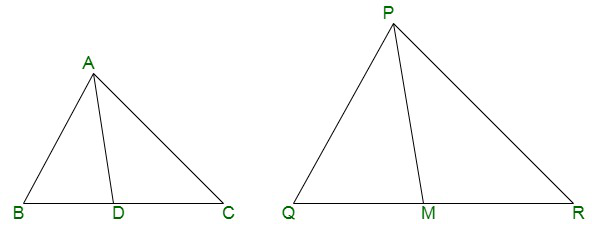Solution:

Given that in ΔABC and ΔPQR,

AB is proportional to PQ

BC is proportional to QR

That is, AB/PQ = BC/QR = AD/PM

We know,

Since, D is the midpoint of BC and M is the midpoint of QR

By SSS similarity criterion, we have,

⇒ ΔABD ~ ΔPQM

Now, since the corresponding angles of two similar triangles are equal, we obtain,

∴ ∠ABD = ∠PQM

⇒ ∠ABC = ∠PQR

Now,

In ΔABC and ΔPQR

AB/PQ = BC/QR ………….(i)

∠ABC = ∠PQR ……………(ii)

From equation (i) and (ii), we get,

By SAS similarity criterion, we have,

ΔABC ~ ΔPQR

### Question 13. D is a point on the side BC of a triangle ABC such that ∠ADC = ∠BAC. Show that CA2 = CB.CD

Solution:

Given, D is a point on the side BC of a triangle ABC such that ∠ADC = ∠BAC.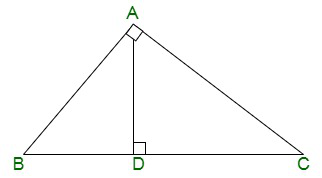∠ACD = ∠BCA (Common angles)

By AA similarity criterion, we have,

Since, the corresponding sides of similar triangles are in proportion, we obtain,

∴ CA/CB = CD/CA

That is, CA2 = CB.CD.

Hence, proved.

### Question 14. Sides AB and AC and median AD of a triangle ABC are respectively proportional to sides PQ and PR and median PM of another triangle PQR. Show that ΔABC ~ ΔPQR.

Solution:

Given, Two triangles ΔABC and ΔPQR in which AD and PM are medians such that;

Now,

Construction,

Produce AD further to E so that AD = DE. Now, join CE.

Similarly, produce PM further until N such that PM = MN. Join RN.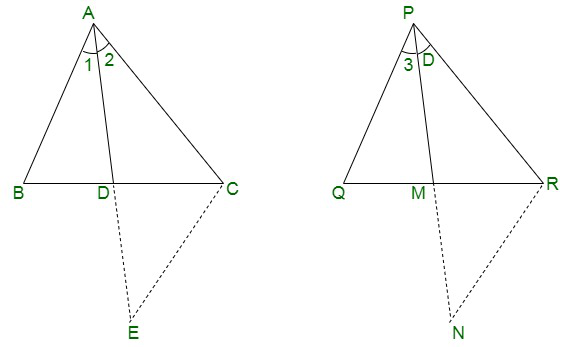In ΔABD and ΔCDE, we have

From the construction done,

Now, since AP is the median,

BD = DC

and, ∠ADB = ∠CDE [Vertically opposite angles are equal]

By SAS criterion,

∴ ΔABD ≅ ΔCDE

By CPCT, we have,

⇒ AB = CE……………..(i)

In ΔPQM and ΔMNR,

From the construction done,

PM = MN

Now, since PM is the median,

QM = MR

and, ∠PMQ = ∠NMR [Vertically opposite angles are equal]

By SAS criterion,

∴ ΔPQM = ΔMNR

By CPCT,

⇒ PQ = RN …………………(ii)

Now, AB/PQ = AC/PR = AD/PM

From equation (i) and (ii), we conclude,

⇒ CE/RN = AC/PR = 2AD/2PM

We know,

2AD = AE and 2PM = PN.

⇒ CE/RN = AC/PR = AE/PN

By SSS similarity criterion,

∴ ΔACE ~ ΔPRN

Thus,

∠2 = ∠4

And, ∠1 = ∠3

∴ ∠1 + ∠2 = ∠3 + ∠4

⇒ ∠A = ∠P ………………….(iii)

Now, in ΔABC and ΔPQR, we have

AB/PQ = AC/PR [Given]

From equation (iii), we have,

∠A = ∠P

By SAS similarity criterion,

∴ ΔABC ~ ΔPQR

### Question 15. A vertical pole of a length 6 m casts a shadow 4m long on the ground and at the same time a tower casts a shadow 28 m long. Find the height of the tower.

Solution:

Given, Length of the vertical pole = 6m

Length of shadow of the tower = 28 m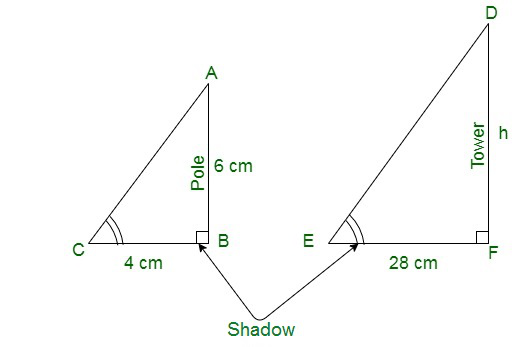Shadow of the pole = 4 m

Let us assume the height of tower to be h m.

In ΔABC and ΔDEF,

∠C = ∠E (By angular elevation of sum)

Since, the following angles are equivalent to 90°

∠B = ∠F

By AA similarity criterion, we have,

∴ ΔABC ~ ΔDEF

Since, if two triangles are similar corresponding sides are proportional

∴ AB/DF = BC/EF

Substituting values,

∴ 6/h = 4/28

⇒h = (6×28)/4

⇒ h = 6 × 7

⇒ h = 42 m

Hence, the height of the tower specified is 42 m.

### Question 16. If AD and PM are medians of triangles ABC and PQR, respectively where ΔABC ~ ΔPQR prove that AB/PQ = AD/PM.

Solution:

Given, ΔABC ~ ΔPQR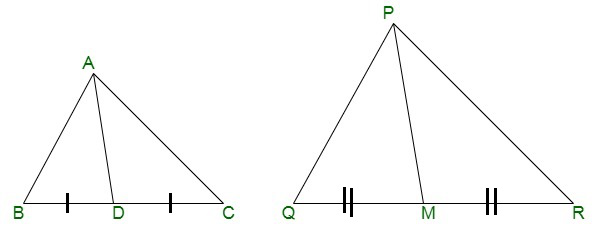Since, the corresponding sides of similar triangles are in proportion.

∴ AB/PQ = AC/PR = BC/QR……………(i)

And, ∠A = ∠P, ∠B = ∠Q, ∠C = ∠R ………….…..(ii)

Since, AD and PM are the medians, they will divide their opposite sides correspondingly.

∴ BD = BC/2 and QM = QR/2 ………….(iii)

From equations (i) and (iii), we obtain,

AB/PQ = BD/QM …………….(iv)

In ΔABD and ΔPQM,

From equation (ii), we have

∠B = ∠Q

From equation (iv), we have,

AB/PQ = BD/QM

By SAS similarity criterion, we have,

∴ ΔABD ~ ΔPQM

That is, AB/PQ = BD/QM = AD/PM

My Personal Notes arrow_drop_up
Related Tutorials